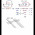## Wednesday, June 19, 2013

### Problem of the Week Day 3: Week of 6/17/13 - 6/21/13

Today is day three of the problem of the week! Monday was trigonometry, Tuesday was algebra, so we will make Wednesday be number theory. This branch of math might sound foreign to you, but the concepts within it that I plan to use in the problems have all been taught to you at some point in your life.

Easy:
Find the Greatest Common Factor of 100y and 100z. We will call this number g.

g = _____

Medium:
What is the number of dots in a regular t-gon array whose sides are of length h? In other words, look at the figurative families, and find the tth family. Then, find the hth number in that family. Call that number n.

n = _____

Hard:
Take the following sequence:

x1x2, p + y1, (q + 110x+ 4)/(t), q– q– q10 – q11 – q12 – q13

Now, find the explicit formula for this sequence. For the simplicity of the variables, I will tell you that the formula should be a quadratic function. Use the ax2 + bx + c model, with a, b, and c being the answers to this problem. They are also the coefficients of the equation.

a = _____
b = _____
c = _____

Good luck!

1.2.